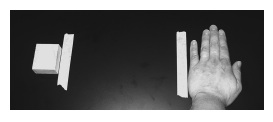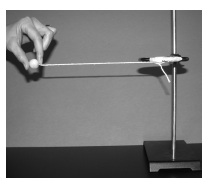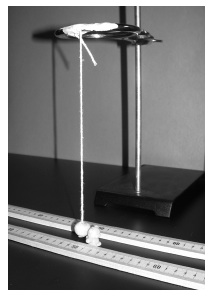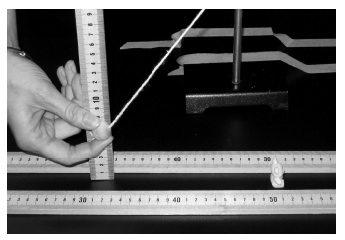# Investigation 1 – Lab

### MINDSETThis Investigation is designed to:

• introduce you to kinetic and potential energy,
• demonstrate the dependence of kinetic energy on velocity,
• illustrate the dependence of potential energy on height,
• provide opportunities for you to use formulas and data in calculations, and
• promote your understanding of the Law of Conservation of Energy.

### BE PREPARED

Student Preparation for the Investigation includes having students gather the following materials. This preparation takes place on lab day after student lab groups have settled at their assigned lab tables.

Note: The materials are listed in students’ SDRs. They are also listed below for your reference.

• (1) triple beam balance
• (1) ring stand with ring
• (1) 35 cm piece of string
• (1) pendulum ball
• (1) small bear
• (3) meter sticks
• (1) clear metric ruler
• (1) woodblock
• scissors
• (1) calculator

Direct one student from each lab group to collect the materials listed in their SDRs.

### INVESTIGATE

• The first type of energy you will investigate is kinetic energy.
• Kinetic energy is the energy of motion. All objects that are moving have kinetic energy.
• Reflect on the PreLab video as you move through the procedural steps.
• During the Experiment, every procedural step is important. If one step is skipped, data can become invalid. To help you keep on track, read each step thoroughly, complete the step, then check it off (Read it – Do it – Check it).
• Complete all of the procedural steps in your SDR.

Note: The procedural steps are listed below for your reference.

1. Put one piece of masking tape on the lab table approximately 15 cm from the edge. Place a second piece of masking tape 20 cm away from the first piece.
2. Place a wood block beside the second piece of tape.
3. Place your hand flat on the table behind the piece of tape next to the edge of the table.Note: As you perform the trials with the woodblock, be sure that you are not pushing the block. The hand should not accelerate. Instead, it should move at a constant speed and stop after hitting the block.

Trial 1:
1. In this Trial, you will move your hand slowly across the lab table until it hits the woodblock. Make sure your hand stops moving after it hits the block.
2. How far did the block move? Measure the distance from the tape to the block. Use proper units.

Trial 2:

1. In this Trial, you will move your hand quickly across the table until it hits the woodblock. Again, make sure your hand stops moving as it hits the woodblock.
1. Place the wood block back beside the piece of tape.
2. Repeat the experiment.
2. How far did the block move? Measure the distance from the tape to the block. Use proper units.
• After you have performed the trials with the woodblock and answered the questions, consider the following:
• You should understand that objects that are moving have kinetic energy.
• You should have observed that kinetic energy can be transferred from one object to another (hand to block).
• You should also have discovered the relationship between the amount of kinetic energy and the distance the block moved. As the kinetic energy increased, the distance the woodblock traveled increased.
• Do you think an object has to be moving to have energy?
• Read the Background located in Trial 2 of the Student Data Record.
• One type of potential energy (gravitational potential energy) depends on the height of an object above a surface.
• Next, you will be experimenting with this type of potential energy in the remainder of the Investigation.
1. Thread the piece of string through the hole in the pendulum ball. Tie a knot at the bottom of the string. Cut off any excess string below the knot.
2. Place a ring on the ring stand. Clamp the ring onto the support rod so that the ring is over the table, not over the base.
3. Attach the pendulum ball string to the ring stand. Use masking tape to make sure the string does not move on the ring.
4. Adjust the ring on the support rod so that the bottom of the ball is approximately 1 cm above the surface of the table. Be sure that the knot does not touch the table.
5. Pull the pendulum ball up and to the side so that it is level with the ring. Hold the ball in that position. Carefully lower the ball.
6. Repeat step 7, but this time let go of the ball.

Note: Step 7 is step 13 in your SDR due to the questions integrated into the procedures.

Note: Make sure that you construct your pendulums with the ball hanging over the table surface and not over the base of the ring stand. If you are having difficulty, look at the pictures in steps 9-13 of Trial 2 in your Student Data Record as you set up the experiment.

Note: When you are dropping the pendulum ball, be sure you just let go and do not push the ball.Optional Extension: This portion of the Investigation can be extended, if your teacher thinks that there is sufficient time, by introducing the equation for kinetic energy: KE = ½ mv2, where m is the mass of the object, and v is the velocity. You could calculate the velocity of the hand by dividing the distance the hand traveled (20 cm or 0.2 m) by the time it took to cover the distance. Although the mass of the hand cannot be determined, the velocity for both trials can be entered into the equation to show mathematically that kinetic energy is higher at the faster velocity.

• After you have answered Questions 14-20, discuss the potential energy in the experiment.
• You should understand that the ball had only potential energy when it was being held. It had potential energy because it was raised to a height above its rest position. The potential energy was converted to kinetic energy when the ball was let go and began to move.
• The ball had potential energy at all points except when it was at the bottom of the pendulum arc. This is the zero position for height, so there is no potential energy at this point.
• Now, set up the pendulum apparatus:
1. Place two meter sticks lengthwise on the table and parallel to each other. The meter sticks should be 3 cm apart, and the zero ends should be lined up.
2. Tape the meter sticks to the table at both ends so that they do not move.
3. Place the gram bear between the meter sticks. The back of the bear should line up with the 50 cm marks on the meter sticks.
4. Move the ring stand so that the base is outside of the meter sticks and the pendulum ball is hanging behind the bear. The pendulum ball and the gram bear should be touching. The string on the pendulum ball should be going straight down.
5. Tape the ring stand base to the table so that it does not move.Note: Be sure that the back of the bear is at the 50 cm mark and that the pendulum ball is hanging straight down and touching the back of the bear.
Note: You may want to take a few practice swings before proceeding with Trial 3.
Note: The pendulum ball should be pulled straight back before releasing it.

Trial 3
1. In this Trial, you will investigate the effect of swinging the pendulum from a height of 5 cm.
1. Without moving the bear, pull the ball back to a height of 5 cm. Use a meter stick to measure the height from the table surface to the bottom of the ball.
2. Let go of the pendulum ball.1. Calculate: Measure the distance the bear traveled.
1. If the bear fell down, measure from the point of the bear closest to the ball. It may help to place a ruler at the edge of the bear, across the two meter sticks.
2. The bear started at 50 cm, so subtract 50 cm from the measurement to see how far the bear traveled.
2. Record: Write the distance the bear traveled next to “Run A” in Table A.
3. Repeat the experiment two more times with the ball at 5 cm.
4. Record: Write the distance the bear traveled in Runs B and C in Table A.
5. Calculate: Find the average distance the bear traveled. To find the average distance, add the three measurements together and divide by three. Write the average in the last row of Table A.

Trial 4
1. In Trial 4 you will repeat the experiment, but in this trial, you will pull the ball back to a height of 10 cm. As before, take three measurements with the ball at 10 cm.
2. Record: Write the distance the bear traveled in each run in Table B.
3. Calculate: Find the average distance the bear traveled. To find the average distance, add the three measurements together and divide by three. Write the average in the last row of Table B.

Trial 5
1. In this Trial, you will repeat the experiment, but this time you will raise the ball to a height of 20 cm. Take three measurements with the ball at 20 cm.
2. Record: Write the distance the bear traveled in each run in Table C.
3. Calculate: Find the average distance the bear traveled. To find the average distance, add the three measurements together and divide by three. Write the average in the last row of Table C.

• As a class, read the Background following Table C in the Student Data Record.
• Consider the equation for potential energy.

Potential Energy (mJ) = mass of ball (g) × (9.81 m/s2) × height (m)

1. Remove the pendulum ball from the string.
2. Use the Procedure, Triple Beam Balance Use and Operation, to determine the mass of the pendulum ball.
3. Record: What is the mass of the pendulum ball in grams?
4. Calculate: Change the height of the ball (5 cm, 10 cm, and 20 cm) to height in meters. To find height in meters, divide the height in centimeters by 100.
5. Record: Write each height of the pendulum ball in meters in Table D.
6. Calculate: Use the potential energy equation to determine the potential energy of the pendulum ball at each height.
Potential Energy (mJ) = mass of ball (g) × (9.81 m/s2) × height (m)
1. Record: Write the potential energy for each height of the pendulum ball in Table D.
2. Record: Write the average distance the bear traveled after being hit by the ball in Table D. Copy the average distance from Tables A, B, and C.

### CLEAN UP

Be sure to clean up your lab bench after completing your experiments.